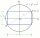# Rectangle + analytic geometry - math problems

#### Number of problems found: 6

• Coordinates of the intersection of the diagonalsIn the rectangular coordinate system, a rectangle ABCD is drawn. The vertices of the rectangle are determined by these coordinates A = (2.2) B = (8.2) C = (8.6) D = (2.6) Find the coordinates of the intersection of the diagonals of the ABCD rectangle
• Rectangle 39Find the perimeter and area of the rectangular with vertices (-1, 4), (0,4), (0, -1), and (-4, 4)
• Set of coordinatesConsider the following ordered pairs that represent a relation. {(–4, –7), (0, 6), (5, –3), (5, 2)} What can be concluded of the domain and range for this relation?4 m long ladder touches the cube 1mx1m at the wall. How high reach on the wall?Show that the quadrilateral with vertices P1(0,1), P2(4,2) P3(3,6) P4(-5,4) has two right triangles.
• A screenA screen is 1680 x 1050 pixels. What are the coordinates (and size in pixels) of an centered area which is exactly 33% of the screen size?

We apologize, but in this category are not a lot of examples.
Do you have an interesting mathematical word problem that you can't solve it? Submit a math problem, and we can try to solve it.

We will send a solution to your e-mail address. Solved examples are also published here. Please enter the e-mail correctly and check whether you don't have a full mailbox.

Please do not submit problems from current active competitions such as Mathematical Olympiad, correspondence seminars etc...

For Basic calculations in analytic geometry is a helpful line slope calculator. From coordinates of two points in the plane it calculate slope, normal and parametric line equation(s), slope, directional angle, direction vector, the length of segment, intersections the coordinate axes etc. Rectangle Problems. Analytic geometry - math word problems.